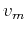Time-to-depth conversion and seismic velocity estimation using time-migration velocityNext: Seismic Velocity Up: Cameron, Fomel, Sethian: Velocity Previous: Introduction

# Time Migration Velocity

Kirchhoff prestack time migration is commonly based on the following travel time approximation (Yilmaz, 2001). Letbe a source,be a receiver, andbe the reflection subsurface point. Then the total travel time fromtoand fromtois approximated as(1)

whereandare effective parameters of the subsurface point. The approximationusually takes the form of the double-square-root equation(2)

whereandare the escape location and the travel time of the image ray (Hubral, 1977) from the subsurface point. Regarding this approximation, let us list four cases depending on the seismic velocityand the dimension of the problem:
2-D and 3-D, velocityis constant.
Equation 2 is exact, and.
2-D and 3-D, velocitydepends only on the depth.
Equation 2 is a consequence of the truncated Taylor expansion for the travel time around the surface point. Velocitydepends only onand is the root-mean-square velocity:(3)

In this case, the Dix inversion formula (Dix, 1955) is exact. We formally define the Dix velocityby inverting equation 3, as follows:(4)

2-D, velocity is arbitrary.
Equation 2 is a consequence of the truncated Taylor expansion for the travel time around the surface point. Velocityis a certain kind of mean velocity, and we establish its exact meaning in the next section.
3-D, velocity is arbitrary.
Equation 2 is heuristic and is not a consequence of the truncated Taylor expansion. In order to write an analog of travel time approximation 2 for 3-D, we use the relation (Hubral and Krey, 1980)(5)

whereis the matrix of the second derivatives of the travel times from a subsurface pointto the surface,is the matrix of radii of curvature of the emerging wave front from the point source, andis the velocity at the surface point. For convenience, we prefer to deal with matrix, which, according to equation 5 is(6)

The travel time approximation for 3-D implied by the Taylor expansion is(7)The entries of the matrixhave dimension of squared velocity and can be chosen optimally in the process of time migration. It is possible to show, however, that one needs only the values of(8)

to perform the inversion. This means that the conventional 3-D prestack time migration with traveltime approximation 2 provides sufficient input for our inversion procedure in 3-D. The determinant in equation 8 is well approximated by the square of the Dix velocity obtained from the 3-D prestack time migration using the approximation given by equation 2.
One can employ more complex and accurate approximations than the double-square-root equations 2 and 7, i.e. the shifted hyperbola approximation (Siliqi and Bousquié, 2000). However, other known approximations also involve parameters equivalent toor.Time-to-depth conversion and seismic velocity estimation using time-migration velocityNext: Seismic Velocity Up: Cameron, Fomel, Sethian: Velocity Previous: Introduction

2013-03-02